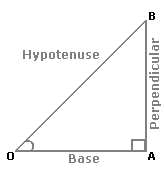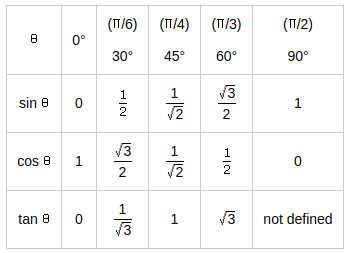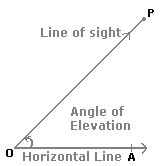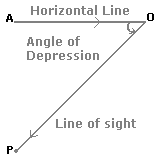## Height and Distance

### introduction to Height and Distance

trignomentry :

In a right-angled$$\triangle$$ OAB, where $$\angle \mathrm{BOA}$$=$$\mathrm{\theta}$$• $$\sin \theta=\frac{\text { Perpendicular }}{\text { Hypotenuse }}=\frac{A B}{O B}$$
• $$\cos \theta=\frac{\text { Base }}{\text { Hypotenuse }}=\frac{O A}{O B};$$
• $$\tan \theta=\frac{\text { Perpendicular }}{\text { Base }}=\frac{A B}{O A};$$
• $$\csc \theta=\frac{1}{\sin \theta}=\frac{O B}{A B}$$;
• $$\sec \theta=\frac{1}{\cos \theta}=\frac{O B}{O A}$$

Trigonometrical Identities:

1. $$\sin ^{2} \theta+\cos ^{2} \theta=1$$
2. $$1+\tan ^{2} \theta=\sec ^{2} \theta$$
3. $$1+\cot ^{2} \theta=\csc ^{2} \theta$$

### Value of T ratios

Value of T rotationsAngle of Elevation:Suppose a man from a point O looks up at an object P, placed above the level of his eye. Then, the angle which the line of sight makes with the horizontal through O is called the angle of elevation of P as seen from O.

$$\therefore$$The angle of elevation of P from O= $$\angle \mathrm{AOP}$$

An angle of Depression:Suppose a man from a point O looks down at an object P, placed below the level of his eye, then the angle which the line of sight makes with the horizontal through O is called the angle of depression of P as seen from O.